##### Actions

(diff) ← Older revision | Latest revision (diff) | Newer revision → (diff)

The words "Braess's paradox" refer to a surprising decrease in performance for some network, which is a result of it being improved locally. The occurrence of this decrease has been studied in mathematical models of equilibrium flow in road/rail traffic, computer networks, telephone networks, water supply systems, electrical circuits, spring systems, and so on. This has been done under various assumptions on routing schemes, such as being state-dependent or fixed.

Below, the occurrence of Braess's paradox in a classical model of user equilibrium traffic flow is given.

Let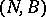be a finite directed graph, with node set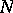and arc, branch or link set(cf. also Graph, oriented). A path in which all links are similarly directed is called a route, with the initial and final nodes forming an origin/destination pair (or O/D pair). One considers a set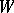of O/D pairs, and for each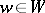, supposes a flow demand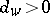to be given. Let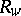be a set of routes joining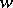. For each, and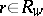, one considers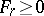such that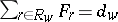, giving a route flow vector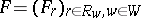. This route flow induces a link flow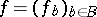, by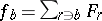for each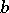, where one identifies a route with the set of its links. For each link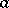, one supposes a link cost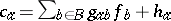, where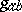and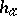are given. For,, one defines a route cost by.

A route flow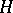is a user equilibrium if it satisfies the condition that for all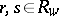,, if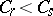then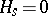. In other words, there is, for each, a common route costfor all routeswith non-zero. A user equilibrium flow exists always [a5], and if the matrix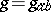is such thatis positive-definite, then the equilibrium link flows, and hence the route costs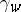, are unique. In [a3], Braess's paradox is said to occur if adding a new routeto someresults inbeing increased. See also [a3] for necessary and sufficient conditions for this to happen under the assumption that there is a strictly positive equilibrium flow in all routes.

There is no fixed definition of Braess's paradox in all systems, but there is a common theme. One assumes some measure of performance,, such as. On the network given by any one link,depends on the flowand a parameter. For example,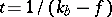if the link is given by an M/M/1 queue (cf. also Queue). Note thatdecreases if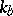increases, forfixed. Suppose some flow demand is fixed and link flows are given by some requirement about equilibria, or by a given dynamical process not in equilibrium. One says that Braess's paradox occurs if, for the network as a whole,increases when someincreases. Adding links may be thought of as changing a parameterfrom zero or infinity. A different language would be used to describe certain other types of networks, such as electrical circuits.

Under this description, the Downs–Thomson paradox [a4] is a particular type of Braess's paradox. If one distinguishes these paradoxes mathematically, it is by requiring the link costsin the Braess paradox to be increasing functions of the link flow, while in the Downs–Thomson paradox there is a link witha decreasing function of.

Independent discoveries of Braess's paradox can be attributed to D. Braess [a1], A. Downs [a4], J.M. Thomson [a6] and C.A. Zukowski and J.L. Wyatt [a7].

[a2] contains an ample list of references.

How to Cite This Entry: